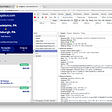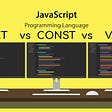# The Prompt

Create a function that takes an array of at least three integers and returns a sorted array of the three largest integers in the input array.

Note: You cannot sort the input array

The function should return duplicate integers, if necessary. For example:

Input array -> [10, 5, 9, 10, 13]

Output -> [10, 10, 13]

# The Code

So we’re given the prompt and the starting code below:

First, we can create a variable to represent the final output array. At this point, the code can’t derive which elements within the input array are the largest, so we’ll initiate our variable with null values. (We will be changing these nulls to integers, as we finalize our code).

Now, let’s initiate a for loop, for each number in the input array. Within this for loop, we’ll create some aspirational code to represent a helper function that will be created to handle changing the values in the largeThree array.

Now, to end this initial function, we simply return the largeThree array and that will be the solution.

Now, it’s time to work on our helper function, in order to finalize the solution and take care of the logic required to give the necessary output.

Now, at this point, since we’re using a helper function. This helper function needs to compare the inputs that are given to it, and then arrange those inputs in a way that will satisfy what the problem is asking of us.

So, in the updateLargest function, we’ll include another helper function within it, that will take care of arranging the integer inputs and output a correct array that will have the three largest integers. The logic will look like the following:

As shown above, updateLargest is taking care of the initial logic, which is to make the comparison between the given inputs.

We’ll create the shiftAndUpdate helper function, so that it may take care of the arranging.

Now, let’s start working on the shiftAndUpdate function.

The final helper function will be traversing through its input array, and if the current element is in the position that is equal to the given index, then that element will be set to num (the input of the helper function). The logic should look like the following:

And that’s it, the final code should look like the following:

# Complexity Analysis

The time complexity of this solution is O(n) time, where (n) is the length of the input array.

The space complexity of this solution is O(1) space.

--

--

--

## More from Karan S. Chauhan

Love podcasts or audiobooks? Learn on the go with our new app.

## Creating a Megabus “Scraper” with Node## How to Use Attribute Directives to Avoid Repetition in Angular Templates## Are You Interested In Tending Node JS ?## The concept of recursion## Basics of JavaScript## NodeConf Budapest 2017## Difference between var vs let vs const## How to Setup GitHub “Pages” and Shave Hours Off of Project-Prep